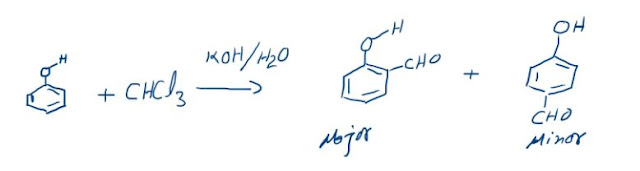## INTERNAL ENERGY OF A SYSTEM

The sum total of all the components of energy due to internal factors of a system is called “Internal Energy” represented by “U”.
It includes the potential energy that is energy of interaction if any and the kinetic energy of the molecules of the system. When we say energy due to internal factors we mean molecular factors. That is we will not include the kinetic energy due to a system’s motion as a whole but will include its molecular kinetic energy. For example when we talk about an ideal gas its molecules are in a continuous and random motion and possess a kinetic energy equal to 3/2 nRT, this becomes the internal energy of this gas. Potential energy is energy of interaction which will be absent when there are no interactions. Internal energy is a state function that is it depends only on the state of the system and not on how the state was attained. It depends only on the state variables. For an ideal gas, it depends only on the absolute temperature for a given sample. For example, for a monoatomic gas:
U = 1.5 n RT
U = f (T)
That is U of an ideal gas will change only on changing the temperature. It may change on changing the pressure as well provided change in pressure results in change of temperature as well which may not be the case always. If we want to calculate the change in internal energy in a process then we have to simply subtract the initial value from the final value as it is a state function.
U = UFINAL - UINITIAL
This relationship will apply to any state function.

### The Reimer-Tiemann reaction: Reaction and its Mechanism

Reimer-Tiemann Reaction Phenols on reaction with chloroform in the presence of sodium hydroxide (or potassium hydroxide) solution give hydro...